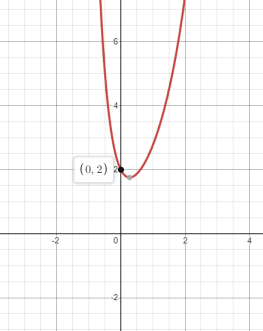# Thevertical and horizontal asymptote of the function.### Single Variable Calculus: Concepts...

4th Edition
James Stewart
Publisher: Cengage Learning
ISBN: 9781337687805### Single Variable Calculus: Concepts...

4th Edition
James Stewart
Publisher: Cengage Learning
ISBN: 9781337687805

#### Solutions

Chapter 4, Problem 13RE

(a)

To determine

## To find: Thevertical and horizontal asymptote of the function.

Expert Solution

y=0 is the Horizontal asymptote and there is no vertical asymptote in the function.

### Explanation of Solution

Given: y=ex+e-3x .

Concept used:

If the degree of the numerator is more than the denominator, there is no horizontal asymptote.

If the denominator has no zeroes then there has no vertical asymptotes

Or

To get Vertical asymptote function should be rational and denominator must contain some variable otherwise there has no vertical asymptote.

Exponential function has horizontal asymptote.

Calculation:

Accordingto the laws of asymptotes:

If the degree of the numerator is more than the denominator, there is no horizontal asymptote.

If the denominator has no zeroes then there has no vertical asymptotes.

The equation of horizontal asymptote is y=0 .

Hence, y=0 is the Horizontal asymptote and there is no vertical asymptote in the function.

(b)

To determine

### To find: TheInterval of increasing or decreasing of the function.

Expert Solution

The Interval of increasing or decreasing of the function is

(,3),(3,0),(0,3) .

Decreasing at interval of (,3) .

Increasing at interval of (3,0),(0,3) .

### Explanation of Solution

Given: y=ex+e-3x .

Concept used:

Increasing or decreasing function can be calculated by equating first derivative of the function to 0.

dydx=0 .

Zeroes of x can be calculatedafter that the increasing and decreasing can be measured.

Calculation:

Increasing or decreasing function can be calculated by equating first derivative of the function to 0.

dydx=0 .

dydx=ex-3e-3x.

ex-3e-3x=0.

logex=log3e-3x.

xloge=3xlog3e

x(loge+3loge)=0 .

x=0 .

Hence the Interval of increasing or decreasing of the function is

(,0) and (0,). .

Decreasing at interval of (,0) .

Increasing at interval of (0,) .

(c)

To determine

### To find: The Local maxima and minima of the function.

Expert Solution

x=0 and y=2 are the point of local minima and there is no

Local maxima.

the point of inflection at x=0 .

### Explanation of Solution

Given: y=ex+e-3x .

Concept used:

The local maxima and minima can be calculated by firstly equating the double differentiation to 0.

1. d2ydx2=0.

2.If d2ydx2>0. the concave will be open upward and local minima can be found.

3. d2ydx2<0. the concave will open downward and local maxima can be found.

Calculation:

y=ex+e-3x

d2ydx2=ex+9e-3x.

At x=0 ,

d2ydx2=e(0)+9e-3(0)=1+9=10.

d2ydx2=10.

d2ydx2>0.

Hence, x=0 and y=2 are the point of local minima and there is no

Local maxima.

the point of inflection at x=0 .

(d)

To determine

### To find: The interval of concavity and the inflection point.

Expert Solution

The interval will be (,0), (0,) .

Concave downward in the interval of (,0) .

Concave upward in the interval of (0,) .

These points are point of inflection

x=0.

### Explanation of Solution

Given: y=ex+e-3x .

Concept used:

The second derivative of function is calculated first.

Set the second derivative equal to zero and solve.

Check whether the second derivative undefined for any values of x.

Plot the number on number line and test the regions with the second derivative.

Plug these 3 values for obtain three inflection points.

The graph of y=f(x) is concave upward on those intervals where

y= f (x)>0 .

The graph of y=f(x) id concave downward on thoseintervals where

y= f (x)<0 .

If the graph of y=f(x) has point of inflection then y= f (x)=0 .

Calculation:

d2ydx2=ex+9e-3x.

ex+9e-3x=0

log(ex+9e-3x)=0

x27xloge3x=0

x(127loge3x)=0

x=0 .

By putting the values in the equation.

The interval will be (,0), (0,) .

Hence,

Concave downward in the interval of (,0) .

Concave upward in the interval of (0,) .

These points are point of inflection

x=0,y=2 .

(e)

To determine

### To Sketch:the graph of the function using graphing device.

Expert Solution

Through the graph it’s easily verified the point of local maxima and minima, function is increasing or decreasing, concavity down or up and point of inflection.

### Explanation of Solution

Given: y=ex+e-3x .

Concept used:

Desmos graphing calculator is used her to plot the graph and it can easily verify the maxima, minima and point of inflection etc.

Calculation:

The graph of y=ex+e-3xHence, through the graph it’s easily verified the point of local maxima and minima, function is increasing or decreasing, concavity down or up and point of inflection.

### Have a homework question?

Subscribe to bartleby learn! Ask subject matter experts 30 homework questions each month. Plus, you’ll have access to millions of step-by-step textbook answers!